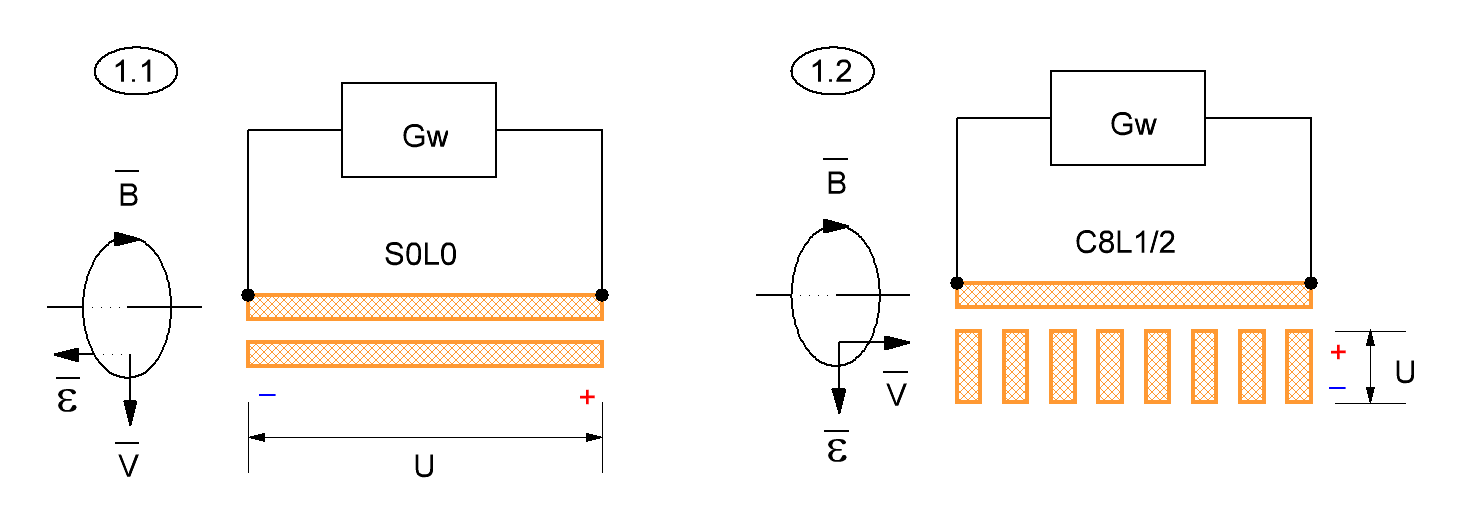Research website of Vyacheslav Gorchilin
2016-10-12
Classification of waves in long lines. Optimum energy extraction
In the first part of this article we looked at some types of standing waves and the method of calculation of their shift. In the second — gave examples of removal of electric energy to the load. Next, we consider the more General classification of types of waves in a long line (DL) and optimal methods of removal depending on this.
So, we now know at least two types of waves: fixed center of the standing wave and moving. Also, we can choose a method of removing electrical energy depending on this: if the center of the standing wave is stationary, the removable non-magnetic conductor (NMP) is parallel to the transmitting (Fig. 1.1), and if the center of the standing wave moves, the removable NWO are perpendicular to the transmitting (figure 1.2).
In Fig. 1 shows two types of waves, direction of the vectors and methods of removal of electric energy. Both schemes work in a similar way. A long line connected to the generator Gw, current flows, which generates a corresponding magnetic field $$\vec B$$.
Figure 1.1
In the first case the lines of force of this field are shifted perpendicular to the axis of the transmitting NMP depending on the current strength. The speed of this shift is indicated by the vector $$\vec V$$. According to the left-hand rule in this case charges are shifted by vector $$\vec{\mathcal{E}}$$, forming a removable NWO, the potential difference $$U$$.
Figure 1.2
In the second case, the magnetic field lines move both perpendicular and the direction of wave motion (this option we will continue to consider). The speed of this shift is also indicated by vector $$\vec V$$. According to the left-hand rule (Lorentz force) in this case, the charges are shifted by vector $$\vec{\mathcal{E}}$$, forming a removable NWO, the potential difference $$U$$. As you can see, in this case the vector $$\vec V$$ and $$\vec{\mathcal{E}}$$, and removable NWO, rotated 90 degrees from figure 1.1.Fig. 1. The mutual arrangement of the vectors depending on the wave form. Two methods of energy extraction
We can now classify some types of waves depending on the amount of the number of harmonics (pulse shape) formed by the generator Gw (Fig. 1), and the size of the DL. This can be done through some notation:
• the first letter in the type designation — wave sine or cosine series of harmonics: $$S$$ — sine, $$C$$ is a cosine.
• one or more digits for the letter — value of the relative PSV calculated by the formula (1.11) or taken from the table;
• dividing the letter symbol $$L$$ denoting the length of the line;
• after the character size should be a long line: $$0, \frac14, \frac12, \frac34, \frac11$$, etc.
Examples of designations
1) $$S0L0$$ where PSV is equal to zero, and the size of the DL small in comparison to a full wavelength, hence the location of the rented NWO parallel transmitting. Ie is the conventional AC transformer (figure 1.1).
2) $$S1L1/4$$ where PSV is equal to one, and the size of the DL — quarter-wave. We already knowthat these parameters has a Tesla transformer. Interestingly, if the excitation is produced by a series of pulses, the number of harmonics of sine and then the first letter of the symbol is $$S$$, and if the excitation occurs in one powerful momentum, then $$C$$. Eat here can be made both parallel and perpendicular to the NWO there; this type of wave can be called a transition.
3) $$C8L1/2$$ where PSV is equal to eight, and the size of the DL — half-wave. In this case, the best would be to eat perpendicular to the NRM (figure 1.2). This design of the device and was proposed in the previous part of the article.
4) $$C100L$$ where PSV is equal to a hundred, and the size of the DL is unknown. The latter figure is not, therefore. This can be, for example, a coaxial cable, which forms a short pulse.
5) $$CXL$$ where PSV is unknown, but the first character indicates rapid growth in passing for impulse. Sizes FOR is also unknown. Therefore, instead of the second digit is a symbol $$X$$ and the last digit is not assigned.
6) $$CXL + S0L0$$ — pair designation is used for devices with different types of waves. In this case means the application of transverse and longitudinal waves in one design. Such a principle is proposed, for example, here.
This klassifikaciya convenient because in addition to wave parameters, and therefore the generator, it automatically suggests the best options for energy extraction. I.e., the frequency of the first harmonic of the generator wave type to fully define the circuitry and design of the entire device.